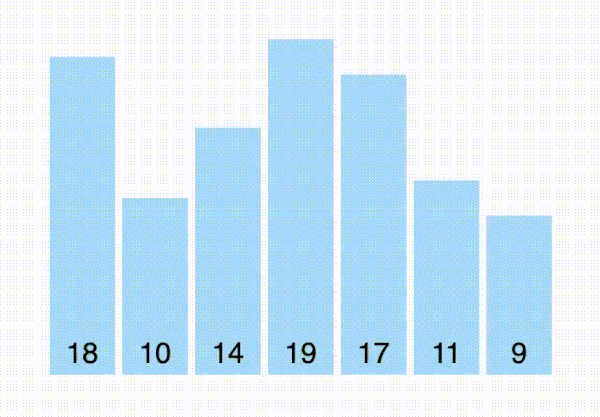Sorting | Skilled.dev
Learn

# Sorting

Sorting is an incredibly important concept in computer science, and many academics devote their career to the topic. In interviews, being told data is either sorted or unsorted is often a key piece of information in a problem. It is not common to need to implement sorting from scratch in an interview, but it is helpful to understand some of the more popular options at a high level.

The most important points to understand for sorting:

1. Sorting is assumed O(n log n)
2. Know how to use the native sorting functionality in your language of choice
3. Understand the implications of sorted vs. unsorted data
4. Special case of O(n) for bucket sort

## Sorting in Interviews

### 1. Sorting is Assumed O(n log n)

It is up to your language or even the implementation of the language to decide which sorting algorithm to use. However, you can assume it will use a O(n log n) version like quick sort or merge sort.

For your interviews, it is unlikely (although not impossible) that you will need to know how to write a sorting algorithm from scratch. If you use a built-in sort function, you'll just need to acknowledge that your sorting will add O(n log n) time complexity.

### 2. Sort in Your Chosen Language

You need to be able to sort for any data type in your language. This could be arrays of numbers, strings, or even objects.

`` ``

### 3. Understand the Implications of Sorted vs. Unsorted Data

If you are told the data is already sorted, then this is often an indication you can use the information to improve on your time complexity, with a very common way being binary search.

If the data is unsorted, then applying a sort to it can improve on a O(n2) or worse solution. Sorting the data can also make it more logical and easier to work with.

### 4. Bucket Sorting

When the data take a fixed range of values, it can be divided into pre-defined buckets, and in this case you can actually sort in O(n) time. You perform one pass over the data to fill your buckets, and then one additional pass over the buckets to form your output.

This is similar to radix sort, but bucket sort is a little more simple to conceptualize and implement. Creating simple buckets is likely all you will need in an interview.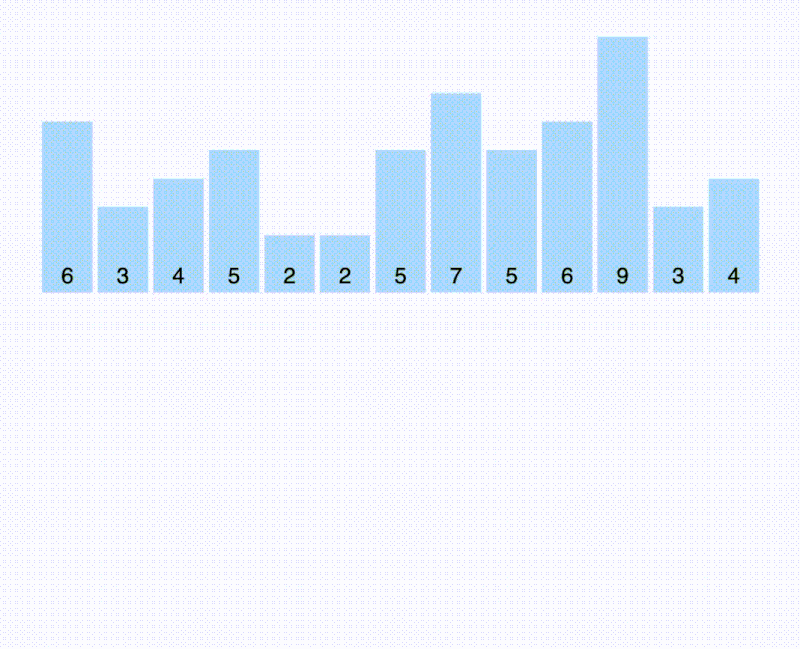## Popular Sorting Algorithms

It's helpful to understand which algorithms have the best runtimes and how they work to gain some intuition for sorting. Below are some of the most popular sorting algorithms. We mention the average and worst case because the arrangement of the items in the array can have a large impact on the runtime, but you are still safe to assume it's O(n log n) for your native sort implementation.

The GIFs below are from VisuAlgo, and another good visualization tool was created by Toptal.

### Selection Sort

Average & Worst Time: O(n2) | Space: O(1)

Selection sort is very inefficient but the easiest to understand. In nested loops, you find the smallest element and move it to the front. Then you find the second smallest and move it to the second spot. Then you find the third smallest and move it to the third spot. You repeat this until all the items are sorted.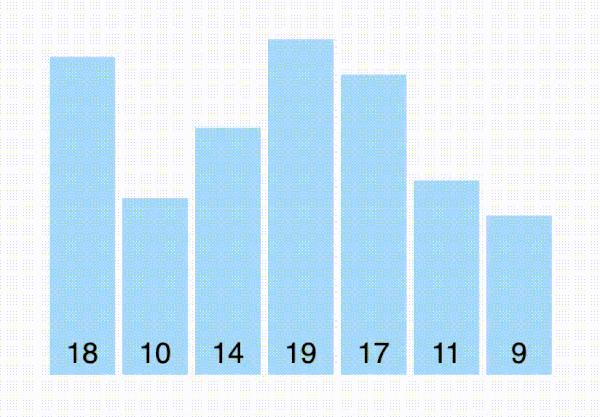### Bubble Sort

Average & Worst Time: O(n2) | Space: O(1)

Bubble sort is also very simple. It iterates through the array and compares each element with the item after it and swaps them accordingly based on their value. It will take O(n2) passes over the input data structure to get it entirely sorted.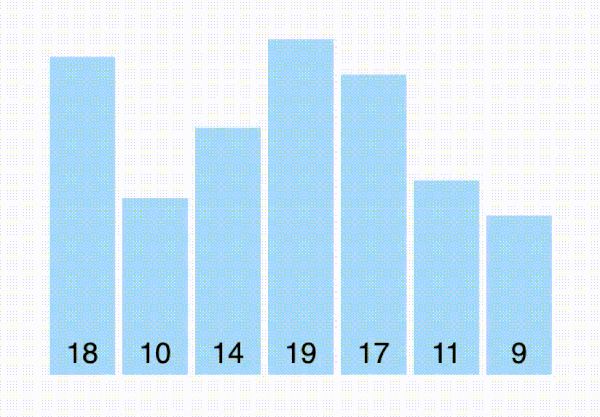### Merge Sort

Average & Worst Time: O(n log n) | Space: O(n log n)

Merge sort is O(n log n), and it is viewed as one of the "good" sorting algorithms. Merge sort repeatedly divides the arrays in half until you reach just two elements. It then sorts these elements and begins merging the arrays back together and sorts them at each merge.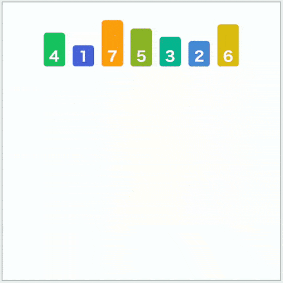### Quick Sort

Average Time: O(n log n), Worst Time: O(n2) | Space: O(n log n)

Quick sort is a "good" sorting algorithm because it operates at O(n log n). However, in the worst case it can be O(n2) when the partition element is not near the median, but this is often assumed to occur very rarely.

Quick sort works by picking a random element and items are swapped it based on whether they are larger or smaller than the partition element.